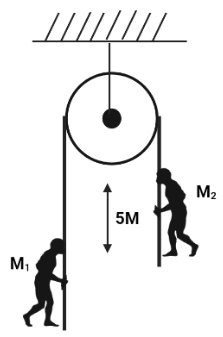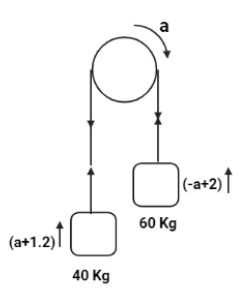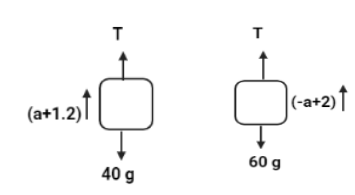Courses
Courses for Kids
Free study material
Free LIVE classes
More# Two men of masses ${m_1}$ and ${m_2}$ hold on the opposite ends of a rope passing over a frictionless pulley. The man ${m_1}$ climbs up the pore with an acceleration of $1.2\,m{\sec ^{ - 2}}$ relative to the rope. The man ${m_2}$ climbs up the rope with an acceleration of $2\,m{\sec ^{ - 2}}$ relative to the rope. They start from rest and are initially separated by 5m. (Given ${m_1} = 40\,Kg$ and ${m_2} = 60\,Kg$).A. Tension in the rope is $555.68\,N$B. Tension in the rope is 556.8 NC. Time after which they will be at the same horizontal level is 1.47 sec.D. Time after which they will be at the same horizontal level is 2.94 sec.

Last updated date: 17th Mar 2023
Total views: 204.9k
Views today: 1.84kVerified
204.9k+ views
Hint:We sketch the point of interest, all forces acting on it, and resolve all force vectors into x– and y–components to create a free-body diagram. Every object in the problem requires a separate free-body diagram.

Let us consider that the acceleration of rope is $a$ which rotates in clockwise direction. Then the acceleration of ${m_1}$ will be $(a + 1.2)$ and the acceleration of ${m_2}$ will be $( - a + 2)$. So, the final diagram is as follows:Now, let us make a free body diagram for the two masses.Calculating the net force we will get two equations.
For the first mass:
$T - 40g = 40(a + 1.2) \\ \Rightarrow T - 40(10) = 40(a + 1.2) \\ \Rightarrow T - 400 = 40a + 48 \\ \Rightarrow T = 40a + 448 \\$
For the second mass:
$T - 60g = 60( - a + 2) \\ \Rightarrow T - 60(10) = 60( - a + 2) \\ \Rightarrow T - 600 = - 60a + 120 \\ \Rightarrow T = - 60a + 720 \\$
Comapring the above two equations we get,
$40a + 448 = - 60a + 720 \\ \Rightarrow 100a = 272 \\ \Rightarrow a = 2.72m.{\sec ^{ - 2}} \\$
Now, lets calculate the value of Tension using this value
$T = - 60a + 720 \\ \Rightarrow T = - 60(2.72) + 720 \\ \Rightarrow T = - 163.2 + 720 \\ \Rightarrow T = 556.8N \\$
Hence, option (B) is correct as Tension in the rope is 556.8N.
Now, we will calculate the individual acceleration of each mass
${a_1} = a + 1.2 \\ \Rightarrow {a_1} = 2.72 + 1.2 \\ \Rightarrow {a_1} = 3.92\,m.{\sec ^{ - 2}} \\$
Similarly,
${a_2} = - a + 2 \\ \Rightarrow {a_2} = - 2.72 + 2 \\ \Rightarrow {a_2} = - 0.72m.{\sec ^{ - 2}} \\$
Now we should calculate the time when both the masses will be in the same horizontal height. Hence, we will use the following formula,
$s = ut + \dfrac{1}{2}a{t^2} \\ \Rightarrow 5 = 0(t) + \dfrac{1}{2}(2.72){t^2} \\ \Rightarrow 10 = 2.72{t^2} \\ \Rightarrow {t^2} = 3.67 \\ \therefore t = 1.48\sec$
Therefore, Option (c) is also correct as time after which they will be at the same horizontal level is 1.47 sec.

Hence, we conclude that option B and option C are the correct answer.

Note:Objects are not often subjected to four powers. The number of forces represented by a free-body diagram can be one, two, or three in some situations. The number of forces that must be drawn in a free-body diagram is not a hard and quick guideline. Drawing free-body diagrams has only one rule: represent all of the forces that occur with that entity in the specified case.